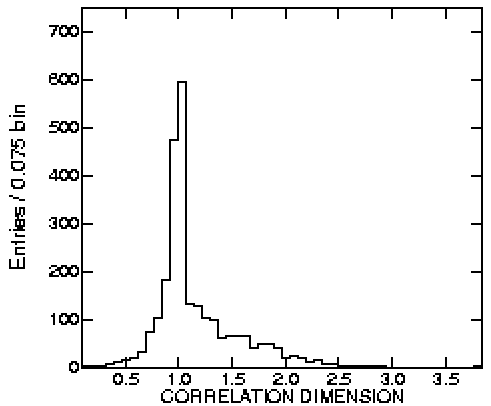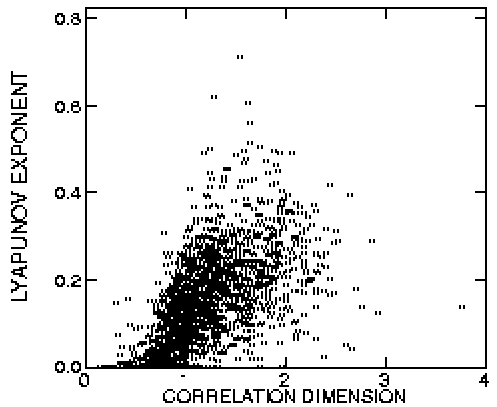# Dynamical Behavior of Artificial Neural Networks with Random Weights

D. J. Albers and J. C. Sprott
Department of Physics, University of Wisconsin, Madison

W. D. Dechert
Department of Economics, University of Houston and Department of Economics, University of Wisconsin, Madison

### ABSTRACT

In this paper we report a Monte Carlo study of the dynamics of large untrained, feedforward, neural networks with randomly chosen weights and feedback. The analysis consists of looking at the percent of the systems that exhibit chaos, the distribution of largest Lyapunov exponents, and the distribution of correlation dimensions. As the systems become more complex (increasing inputs and neurons), the probability of chaos approaches unity. The correlation dimension is typically much smaller than the system dimension.

Ref: D. J. Albers, J. C. Sprott, and W. D. Dechert, in "Intelligent Engineering Systems Through Artificial Neural Networks", Vol. 6, C. H. Dagli, M. Akay, C. L. P. Chen, B. R. Fernandez, and J. Ghosh, eds., ASME Press: New York (1996), pp. 17-22

Fig. 1. Frequency of chaos plot for n = 8, various d and s.Fig. 2. Frequency of chaos plot for s = 8, various d and n.
(An incorrect version of this figure was printed in the paper.)Fig. 3. Lyapunov exponent distribution for n = 8, d = 4, s = 8.Fig. 4. Correlation dimension for n = 8, d = 4, s = 8.Fig. 5. Correlation dimension versus Lyapunov exponent for n = 8, d = 4, s = 8.Computer codes and data used to produce this and related articles are available.